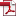• KSII Transactions on Internet and Information Systems
Monthly Online Journal (eISSN: 1976-7277)

# Semi-deterministic Sparse Matrix for Low Complexity Compressive Sampling

Vol. 11, No. 5, May 30, 201710.3837/tiis.2017.05.009, Download Paper (Free):#### Abstract

The construction of completely random sensing matrices of Compressive Sensing requires a large number of random numbers while that of deterministic sensing operators often needs complex mathematical operations. Thus both of them have difficulty in acquiring large signals efficiently. This paper focuses on the enhancement of the practicability of the structurally random matrices and proposes a semi-deterministic sensing matrix called Partial Kronecker product of Identity and Hadamard (PKIH) matrix. The proposed matrix can be viewed as a sub matrix of a well-structured, sparse, and orthogonal matrix. Only the row index is selected at random and the positions of the entries of each row are determined by a deterministic sequence. Therefore, the PKIH significantly decreases the requirement of random numbers, which has a complex generating algorithm, in matrix construction and further reduces the complexity of sampling. Besides, in order to process large signals, the corresponding fast sampling algorithm is developed, which can be easily parallelized and realized in hardware. Simulation results illustrate that the proposed sensing matrix maintains almost the same performance but with at least 50% less random numbers comparing with the popular sampling matrices. Meanwhile, it saved roughly 15%-35% processing time in comparison to that of the SRM matrices.